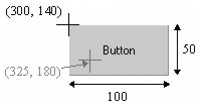DspGetAnFromPoint

Gets the AN of the object at a specified set of screen coordinates. If the X and Y coordinates given are within the extents of an object, then the AN number of the object will be returned.

For example, if there is a button at coordinates (300, 140), and it is 100 wide, 50 high, this function would return the AN if it uses X between 300 & 400 and Y between 140 and 190, such as DspGetAnFromPoint(325,180).Hint: If you are using groups and the specified coordinates point to an object that is part of a group, the AN of the object is returned, not the AN of the group.

Syntax

DspGetAnFromPoint(X, Y [, PrevAN] )

X:

The x coordinate of the screen point.

Y:

The y coordinate of the screen point.

PrevAN:

Retrieves the previous AN (in z-order) in situations where a number of objects overlap at the specified point. The default of 0 (zero) specifies no previous AN. A non-zero value should only ever be passed if it is the result of a previous call to DspGetAnFromPoint.

Return Value

The AN or 0 (zero) if no object exists at the point.

Example

```DspGetMouse(X,Y);
// GetMouse position
AN = DspGetAnFromPoint(X,Y);
// Gets AN if mouse is over the object
Prompt("AN of object ="+AN:###);
!Displays the object's AN at the prompt line
```

If several objects overlap each other at the specified point, the PrevAN argument can be used to produce a list of the associated ANs. This is achieved by using PrevAN to pass the previous result into another call of the function until a zero return is given.

```INT nAn;
nAn = DspGetAnFromPoint(100,100)
WHILE nAn <> 0 DO
//Do Something
nAn = DspGetAnFromPoint(100,100,nAn);
END
```